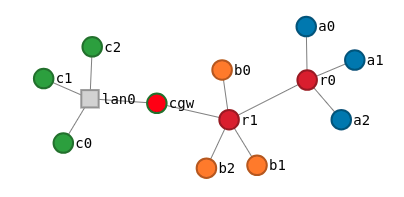This example shows how to connect nodes in interesting ways and how to assign addresses. The topology is composed of 3 enclaves `alpha`, `bravo` and `charlie`. Each enclave is interconnected through a router or a gateway device.### Model Encoded vs Reticulated Addresses#

In this example we programmatically assign addresses to most of the topology and then use the routing reticulator to fill in the rest. A reticulator is a MergeTB element that augments topologies with additional information automatically. This topology is actually using two reticulators:

• `addressing == ipv4`: ensures that all experiment interfaces get an IPv4 address and that all link level neighbors reside on the same subnet.
• `routing == static`: computes a static routing table for the experiment that is set up at materialization time.

The combination of these two reticulators together with the addressing data encoded directly into the model ensure that all nodes in this experiment will be able to talk to each other out of the box at materialization time.

### Complete Example#

import mergexp as mx
from mergexp.unit import mbps, ms
from mergexp.net import capacity, latency, routing, static, addressing, ipv4
# start by creating a topology object
topo = mx.Topology('simple-routes-redux', routing == static, addressing == ipv4)
# it's just python, write your own node constructors
def node(name, group):
dev = topo.device(name)
dev.props['group'] = group
return dev
# node definitions ~~~~~~~~~~~~~~~~~~~~~~~~~~~~~~~~~~~~~~~~~~~~~~~~~~~~~~~~~~~~
alpha = [node(name, 1) for name in ['a0', 'a1', 'a2']]
bravo = [node(name, 2) for name in ['b0', 'b1', 'b2']]
charlie = [node(name, 3) for name in ['c0', 'c1', 'c2', 'cgw']]
routers = [node(name, 4) for name in ['r0', 'r1']]
# connectivity ~~~~~~~~~~~~~~~~~~~~~~~~~~~~~~~~~~~~~~~~~~~~~~~~~~~~~~~~~~~~~~~~
wanlink = topo.connect(routers, capacity == mbps(1000))
# connect nodes
for a in alpha:
topo.connect([a, routers], capacity == mbps(100), latency == ms(10))
for b in bravo:
topo.connect([b, routers], capacity == mbps(5), latency == ms(80))
# connect nodes in 'LAN'
topo.connect(charlie, capacity == mbps(1000), latency == ms(1))
# gateway is special
charlie.props['color'] = '#f00'
dmz = topo.connect(
[charlie, routers],
capacity == mbps(100),
latency == ms(10)
)